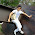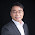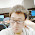## Remove Nth Node From End of List

Given a linked list, remove the nth node from the end of list and return its head.
For example,
   Given linked list: 1->2->3->4->5, and n = 2.

After removing the second node from the end, the linked list becomes 1->2->3->5.

### Updated 201309:More efficient way of solving this problem:

e.g.  1->2->3->4->5->6->7, n=3
Use two pointers:

p
q

(2) head->1->2->3->4->5->6->7     // move q, n elements next to p
p
q
(3) head->1->2->3->4->5->6->7    //  q++ ,p++;
p
q
(4) head->1->2->3->4->5->6->7   //  q++ ,p++;
p
q
(5) head->1->2->3->4->5->6->7   //  q++ ,p++;
p
q
(6) head->1->2->3->4->5->6->7   //  q++ ,p++;
p
q
(7) head->1->2->3->4----->6->7   //  delete p->next
p
q

### Code (Python):

# Definition for singly-linked list.
# class ListNode:
#     def __init__(self, x):
#         self.val = x
#         self.next = None

class Solution:
# @return a ListNode
def removeNthFromEnd(self, head, n):
if n==0 or head==None:
return None

for i in xrange(0,n+1):
if q!=None:
q=q.next
else:
return None

while q:
p=p.next
q=q.next

p.next = p.next.next



### Code(C++):

/**
* Definition for singly-linked list.
* struct ListNode {
*     int val;
*     ListNode *next;
*     ListNode(int x) : val(x), next(NULL) {}
* };
*/
class Solution {
public:
ListNode *removeNthFromEnd(ListNode *head, int n) {
if (n==0 || !head){
return NULL;
}

ListNode* res = new ListNode(0);

for (int i=0;i<=n;i++){
if (q){
q=q->next;
}else{
return NULL;
}
}

while (q){
p=p->next;
q=q->next;
}

p->next=p->next->next;

}
};


Analysis:
This is an easy problem which only needs some basics for pointers.
The idea is to from the first node, scan everyone, check the node's next n node, if the next n node is null, which means meets the end, thus the current node is the nth node from back, then delete the current node, else keep scan the next node.

Some pointer basics:

ListNode *p;
p is a pointer point to one ListNode, *p is the ListNode it pointed to.

new ListNode(0);
returns the pointer to the created instance of ListNode.

Details see the code below.

Code:

/**
* Definition for singly-linked list.
* struct ListNode {
*     int val;
*     ListNode *next;
*     ListNode(int x) : val(x), next(NULL) {}
* };
*/
class Solution {
public:
ListNode *removeNthFromEnd(ListNode *head, int n) {
// Start typing your C/C++ solution below
// DO NOT write int main() function
ListNode *p= new ListNode(0);
while ((p->next)!=NULL){
ListNode *q = p->next;
for (int i=0;i<n;i++){
q=q->next;
}
if (q!=NULL){
p=p->next;
}else{
p->next=p->next->next;
}
}
}
};


1.2.1.